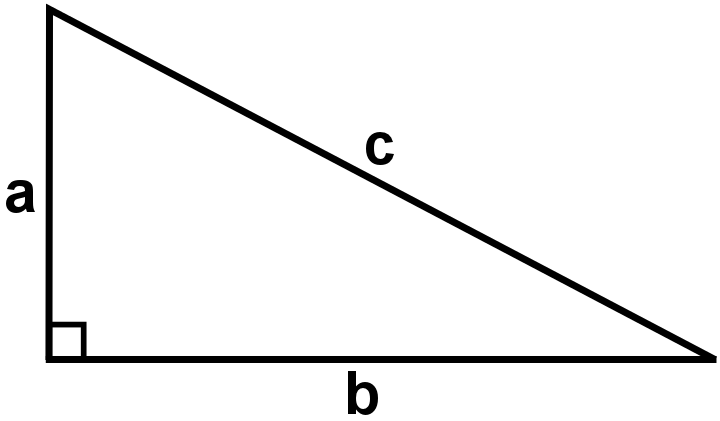#### You may also like### Diophantine N-tuples

Can you explain why a sequence of operations always gives you perfect squares?### DOTS Division

Take any pair of two digit numbers x=ab and y=cd where, without loss of generality, ab > cd . Form two 4 digit numbers r=abcd and s=cdab and calculate: {r^2 - s^2} /{x^2 - y^2}.### Sixational

The nth term of a sequence is given by the formula n^3 + 11n . Find the first four terms of the sequence given by this formula and the first term of the sequence which is bigger than one million. Prove that all terms of the sequence are divisible by 6.

# Pythagoras Perimeters

##### Age 14 to 16Challenge Level

These printable resources may be useful: Pythagoras Perimeters
Pythagoras Perimeters Proof Sorting

### Why do this problem?

This problem offers a challenge in algebraic manipulation, bringing together students' knowledge of area, perimeter, and Pythagoras' Theorem. The Proof Sorter activity offers some scaffolding, so that students can see how to set out a proof, and then use the ideas to construct the proofs that follow on.

### Possible approach"It is possible to show that a right-angled triangle with a perimeter of $12$ units has an area of $36-6c$ square units, where c is the length of the hypotenuse."

"Can you find a way to prove this?"

Give students some time to explore.

They may notice the special case of the $3,4,5$ triangle which satisfies the above conditions, but it is tricky to work out an efficient way to prove it holds for all right-angled triangles with a perimeter of $12$.

Once they have thought about the problem, offer them the Proof Sorter and invite them to put the statements in the correct order.

Once they are happy with the proof, challenge them to adapt their proof to find an expression, in terms of c, for the area of a right-angled triangle with a perimeter of $30$ units.

### Key questions

What equations can you write down about the area and the perimeter of right-angled triangles?
How could you rearrange and manipulate the equations?

### Possible support

Quadratic Patterns offers a good opportunity for students to practise manipulating algebraic expressions in a more accessible context.

### Possible extension

Challenge students to find a general expression, in terms of c, for the area of a right angled triangle with hypotenuse $c$ and perimeter $p$.